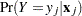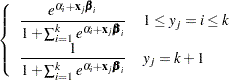### Determining Observations for Likelihood Contributions

If you use the events/trials syntax, each observation is split into two observations. One has the response value 1 with a frequency equal to the value of the events variable. The other observation has the response value 2 and a frequency equal to the value of (trialsevents). These two observations have the same explanatory variable values and the same WEIGHT values as the original observation.

For either the single-trial or the events/trials syntax, letindex all observations. In other words, for the single-trial syntax,indexes the actual observations. And, for the events/trials syntax,indexes the observations after splitting (as described previously). If your data set has 30 observations and you use the single-trial syntax,has values from 1 to 30; if you use the events/trials syntax,has values from 1 to 60.

Suppose the response variable in a cumulative response model can take on the ordered values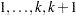, whereis an integer
. The likelihood for theth observation with ordered response value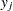and explanatory variables vector ( row vectors)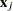is given by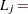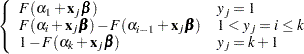where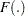is the logistic, normal, or extreme-value distribution function;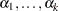are ordered intercept parameters; andis the slope parameter vector.

For the generalized logit model, letting the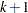st level be the reference level, the interceptsare unordered and the slope vector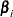varies with each logit. The likelihood for theth observation with ordered response valueand explanatory variables vector(row vectors) is given by